# Excel Advanced Tip: Tell your formulas how to behave with absolute references in formulas using ‘\$’

In Excel, if you write a formula in a cell, it is common for it to contain a cell reference, which means the formula points to a value in one or more other cells.

In the example below, the formula in cell E2 references cells B2, C2 and D2. It determines the total number of phone cards requested during the quarter by adding together the requests in April (B2), May (C2) and June (D2).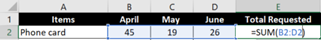But if there are more requested items than phone cards, what is the best way to add a formula to the rows with the other items?

Pro Tip: A formula can be copied down a row or across a column. Left-click in the bottom right corner of cell E2, then continue to hold down the left click until the mouse is dragged down to E7. A formula counting the items in all three months will populate down Column E. This technique will improve your data quality by lessening the chance of errors typing the same formula repeatedly.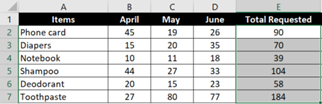Relative Cell References

The most common type of cell reference is a relative cell reference. It is also Excel’s default.

The example above shows relative cell references because the formula in E2 changed its cell references in the different rows of Column E to reflect cells in each row. If you compare the formulas in cells E2 and E7 from the example above, you will notice that the formula is the same except for one small difference. In E2, the formula =SUM(B2:D2) references cells B2, C2 and D2. While in E7, the formula =SUM(B7:D7) references cells B7, C7 and D7.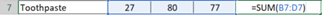Absolute Cell References

Now it’s time to discuss absolute cell references. If a cell contains a formula with an absolute cell reference, that cell reference stays absolutely still. Even if the formula is copied to another cell, the cell reference in the formula will not change. Adding ‘\$’ in front of the column letter and row number of a cell reference will make it an absolute reference.

To show an absolute cell reference, let’s look at what percent of the total requests each item represents.

First, change the style in Column F to the Percent Style. This can be done by right-clicking on the F in Column F to highlight the whole column, then selecting the % button from the Home Ribbon.

To calculate the percent phone cards are of the total requests, see the formula in cell F2, which is =E2/\$E\$9.

• E2 is a relative reference: the cell reference will change if the formula is copied to a new cell.
• \$E\$9 is an absolute reference: Column E and Row 9 are locked with \$s; the cell reference will not change.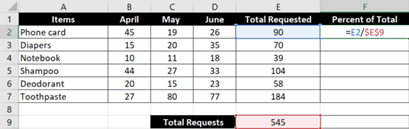Now copy the formula in cell F2 down into the rows of other items in the table. Let’s take a closer look at cell F7. The formula is =E7/\$E\$9.

• E7 is a relative reference: the cell reference changed to reflect the items summed in the row.
• \$E\$9 is an absolute reference: Column E and Row 9 are still locked with \$s; the cell reference did not change even though the row containing the formula did.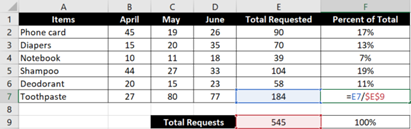You can do a lot with absolute cell references, and we haven’t even talked about the mixed cell references! As you use different types of references, it is important to make sure you understand where formulas used in any given cell are pulling from. To learn more about cell references, check out these two helpful posts about cell references from Microsoft: